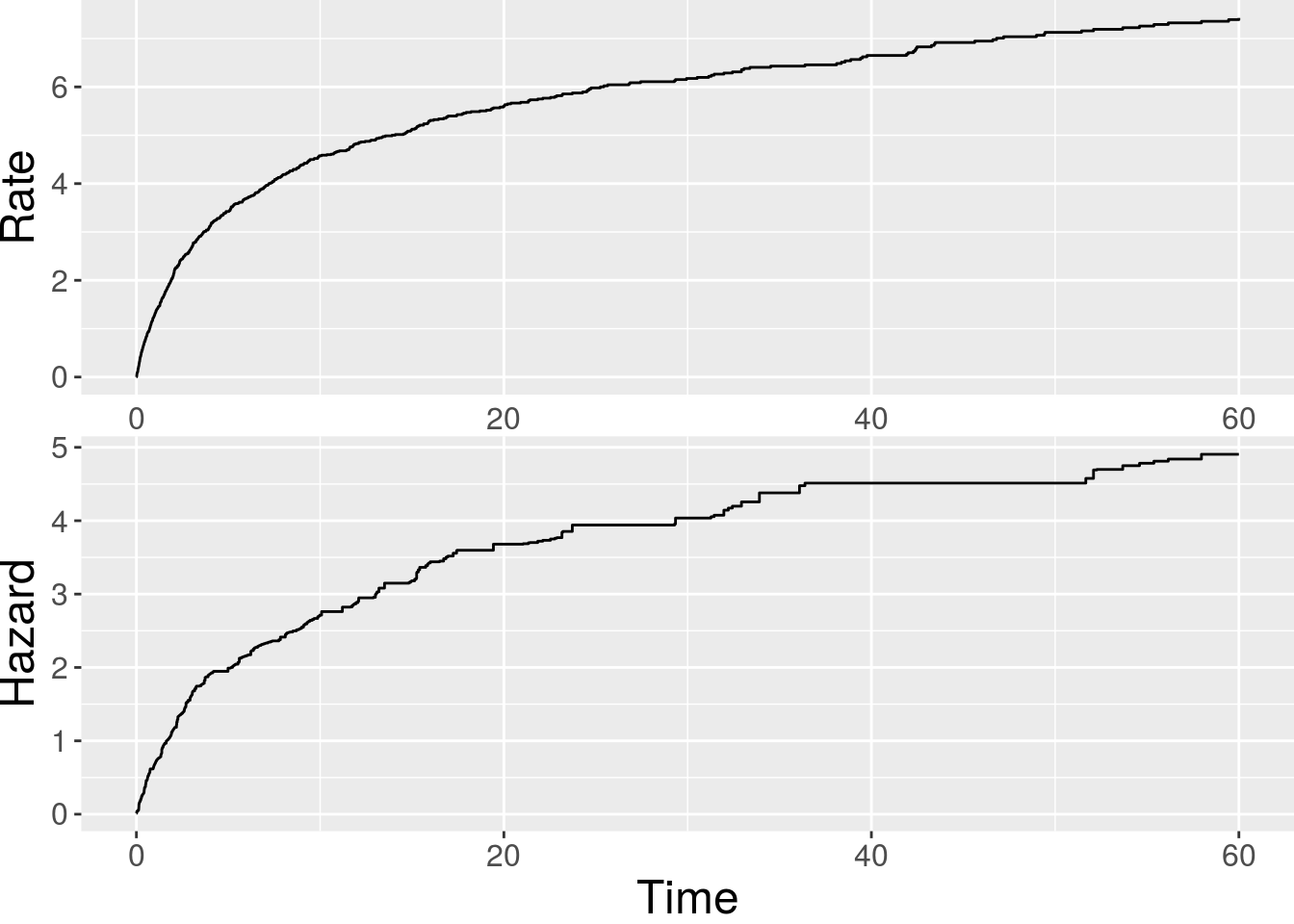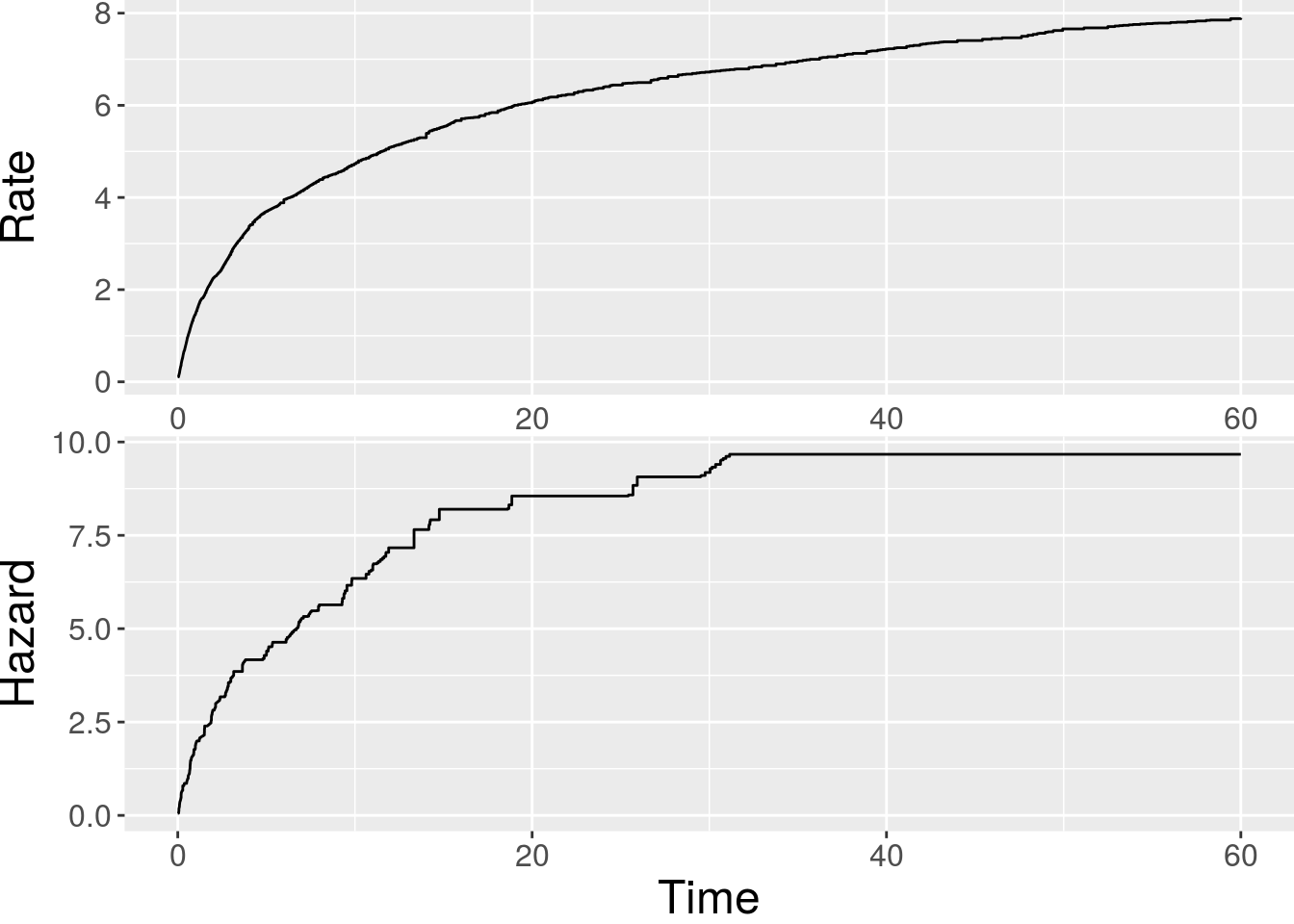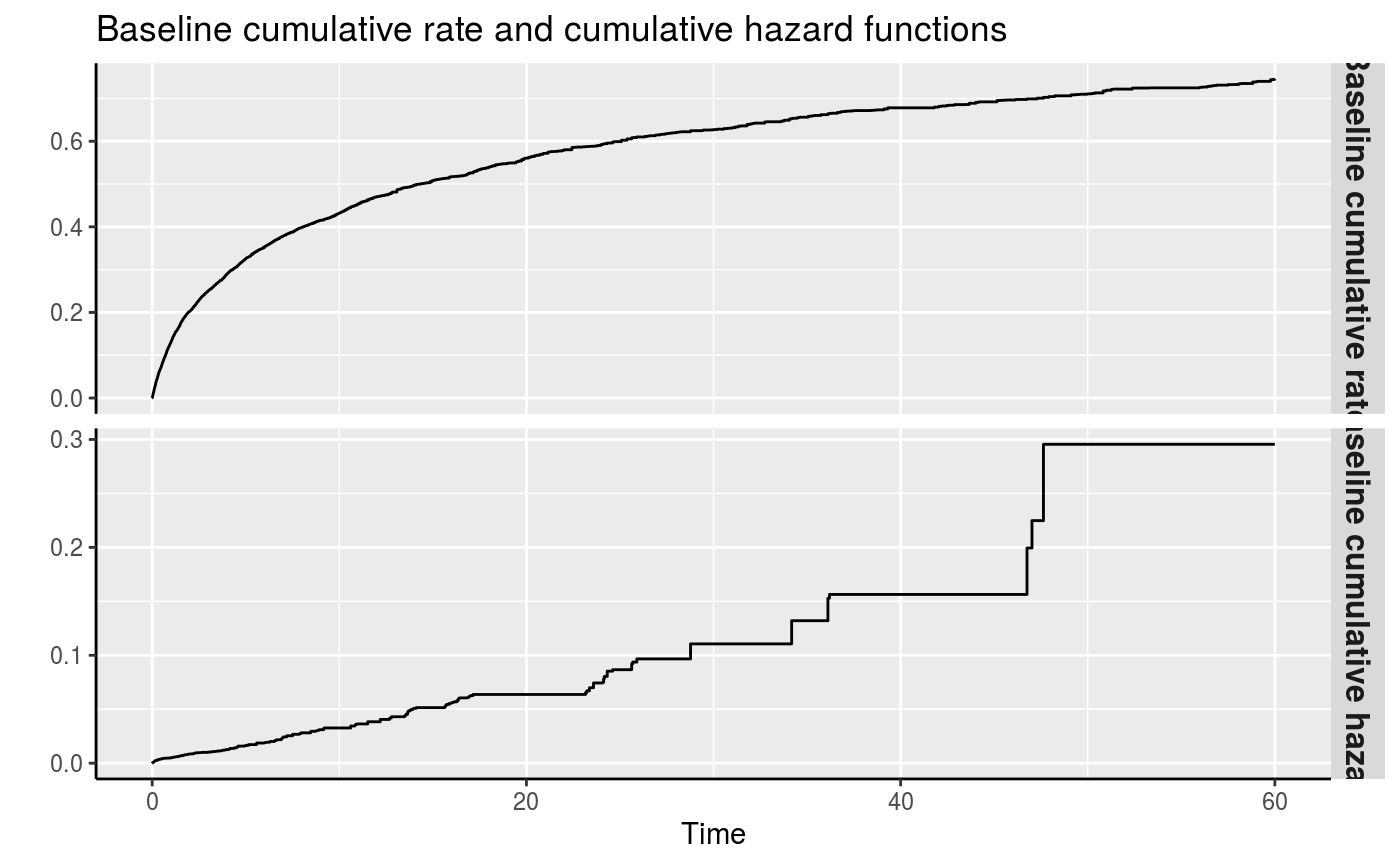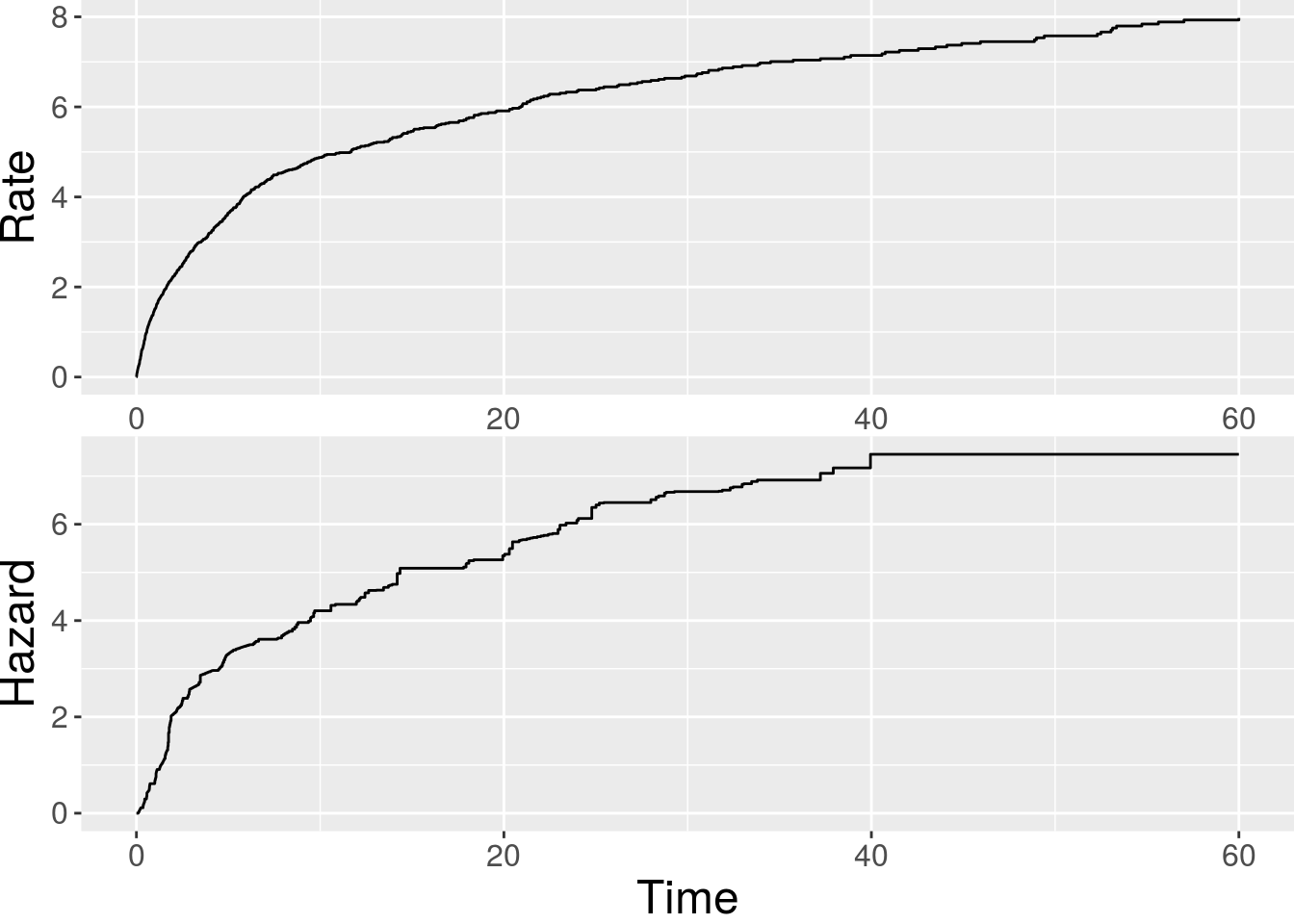In this vignette, we demonstrate how to use the reReg() function in reReg package to fit semiparametric regression models to recurrent event data.

## Model assumption

A general joint model for the rate function of the recurrent event process and the hazard function of the failure time can be formulated as follow: \begin{equation} \lambda(t) = Z \lambda_0(te^{X^\top\alpha})e^{X^\top\beta}; h(t) = Z h_0(te^{X^\top\eta})e^{X^\top\theta}, \label{eq:joint} \end{equation}

where $$Z$$ is a latent shared frailty variable to account for association between the two types of outcomes, $$\lambda_0(\cdot)$$ is the baseline rate function, $$h_0(\cdot)$$ is the baseline hazard function, and the regression coefficients $$(\alpha, \eta)$$ and $$(\beta, \theta)$$ correspond to the shape and size parameters of the rate function and hazard function, respectively. In contrast to many shared-frailty models that require a parametric assumption, following the idea of Wang, Qin, and Chiang (2001), the reReg() function implements semiparametric estimation procedures that do not require the knowledge about the frailty distribution. As a result, the dependence between recurrent events and failure event is left unspecified and the proposed implementations accommodate informative censoring. The reReg() function fits the recurrent event data under the above joint model setting. The arguments of reReg() are as follows

## Arguments of reReg()

> library(reReg); args(reReg)
function (formula, data, B = 200, method = "cox", se = c("resampling",
"bootstrap", "NULL"), control = list())
NULL
• formula a formula object, with the response on the left of a "~" operator, and the predictors on the right. The response must be a recurrent event survival object as returned by function Recur. See the vignette on Visualization of recurrent event data or Introduction to formula response function Recur() for examples in creating Recur objects.
• data an optional data frame in which to interpret the variables occurring in the formula.
• B a numeric value specifies the number of resampling (or bootstrap) for variance estimation. When B = 0, variance estimation will not be performed.
• method a character string specifying the underlying model.
• se a character string specifying the method for standard error estimation.
• control a list of control parameters.

### Choosing model type with method

Model \eqref{eq:joint} includes several popular semiparametric models as special cases, which can be specified via the method argument with the rate function and hazard function separated by "|". For examples, the joint Cox model of Huang and Wang (2004) is a special case of \eqref{eq:joint} when $$\alpha = \eta = 0$$ and can be called by method = "cox|cox"; the joint accelerated mean model of Xu et al. (2017) is a special case when $$\alpha = \beta$$ and $$\eta = \theta$$ and can be called by method = "am|am". Treating the terminal event as nuisances ($$\eta = \theta = 0$$), \eqref{eq:joint} reduces to the generalized scale-change model of Xu et al. (2019), called by method = "sc|.". Moreover, users can mix the models depending on the application. For example, method = "cox|ar" postulate a Cox proportional model for the recurrent event rate function and an accelerated rate model for the terminal event hazard function ($$\alpha = \theta = 0$$ in \eqref{eq:joint}).

### Choosing the variance estimation approach with se

For inference, the reReg() function provides several approaches for variance estimation. The default option is se = "resampling", which refers to the efficient resampling-based sandwich estimator. The general idea is to decompose the limiting covariance matrix in a sandwich form, and its components are estimated via perturbed estimating equations. Details of the resampling approach can be found in Zeng and Lin (2008), Xu et al. (2017), and Xu et al. (2019). The resampling approach is faster than the conventional bootstrap, which can be called with se = "bootstrap", as it only requires evaluating perturbed estimating equations rather than solving them. When se = "bootstrap", user has an option to carry out the bootstrap with parallel computing. This can be done by specifying parallel = TRUE in the control list. When parallel = TRUE, the number of CPU cores can be specified with parCl.

### Control list

The complete control list consists of the following parameters: tol specifies the absolute error tolerance in solving the estimating equations a0, b0 specifies the initial guesses used for root search * solver specifies the equation solver used for root search; the available options are BBsolve, dfsane, BBoptim, and optim (the first three options loads the corresponding equation solver from package BB). * baseSE is an logical value indicating whether the confidence bounds for the baseline functions will be computed. * parallel is an logical value indicating whether parallel computation will be applied when se = "bootstrap" is called. * parCl is an integer value specifying the number of CPU cores to be used when parallel = TRUE. The default value is half the CPU cores on the current host.

## Examples

We will illustrate the usage of reReg with simulated data generated from simSC. Readers are referred to the vignette on Simulating recurrent event data for using simSC to generate recurrent event data.

### Joint Cox model of Huang and Wang (2004)

A simulated model following the joint Cox model of Huang and Wang (2004) can be generated by

> set.seed(1); datCox <- simSC(500, c(1, -1), c(-1, 1), summary = TRUE)

Summary results for number of recurrent event per subject:
Min. 1st Qu.  Median    Mean 3rd Qu.    Max.
0.00    2.00    8.00   18.83   21.00  525.00

Number of failures: 119 (23.8%); Number of censored events: 381 (76.2%)

Number of x1 == 1: 236 (47.2%); Number of x1 == 0: 264 (52.8%)
Summary results for x2:
Min.  1st Qu.   Median     Mean  3rd Qu.     Max.
-3.23639 -0.74943 -0.07149 -0.05930  0.67217  2.68406 

The underlying true model has the form: $\lambda(t) = Z \lambda_0(t)e^{X_1 - X_2}; h(t) = Z h_0(t)e^{-X_1 + X_2}.$ The model fit is

> fit.cox <- reReg(Recur(t.stop, id, event, status) ~ x1 + x2, data = datCox)
> summary(fit.cox)
         Length Class      Mode
alpha        2  -none-     numeric
aconv        1  -none-     numeric
log.muZ      1  -none-     numeric
zi         500  -none-     numeric
Lam0         1  -none-     function
beta         2  -none-     numeric
bconv        1  -none-     numeric
Haz0         1  -none-     function
recType      1  -none-     character
temType      1  -none-     character
alphaSE      2  -none-     numeric
alphaVar     4  -none-     numeric
varMat       9  -none-     numeric
betaSE       2  -none-     numeric
betaVar      4  -none-     numeric
method       1  -none-     character
reTb     59490  -none-     numeric
DF           8  data.frame list
call         3  -none-     call
varNames     2  -none-     character
se           1  -none-     character

The baseline functions can be plotted via plot():

> plot(fit.cox)### Joint accelerated mean model of Xu et al. (2017)

A simulated model following the joint accelerated mean model of Xu et al. (2017) can be generated by

> set.seed(1); datAM <- simSC(500, c(1, -1), c(-1, 1), type = "am", summary = TRUE)

Summary results for number of recurrent event per subject:
Min. 1st Qu.  Median    Mean 3rd Qu.    Max.
0.00    2.00    5.00    6.34    9.00   52.00

Number of failures: 115 (23%); Number of censored events: 385 (77%)

Number of x1 == 1: 236 (47.2%); Number of x1 == 0: 264 (52.8%)
Summary results for x2:
Min.  1st Qu.   Median     Mean  3rd Qu.     Max.
-3.23639 -0.74943 -0.07149 -0.05930  0.67217  2.68406 

The underlying true model has the form: $\lambda(t) = Z \lambda_0(te^{X_1 - X_2})e^{X_1 - X_2}; h(t) = Z h_0(te^{-X_1 + X_2})e^{-X_1 + X_2}.$ The model fit is

> fit.am <- reReg(Recur(t.stop, id, event, status) ~ x1 + x2, data = datAM, method = "am")
> summary(fit.am)
         Length Class      Mode
alpha        2  -none-     numeric
aconv        1  -none-     numeric
log.muZ      1  -none-     numeric
zi         500  -none-     numeric
Lam0         1  -none-     function
beta         2  -none-     numeric
bconv        1  -none-     numeric
Haz0         1  -none-     function
recType      1  -none-     character
temType      1  -none-     character
alphaSE      2  -none-     numeric
alphaVar     4  -none-     numeric
varMat       4  -none-     numeric
betaSE       2  -none-     numeric
betaVar      4  -none-     numeric
method       1  -none-     character
reTb     22020  -none-     numeric
DF           8  data.frame list
call         4  -none-     call
varNames     2  -none-     character
se           1  -none-     character

The baseline functions can be plotted via plot():

> plot(fit.am)### Xu et al. (2019)sc.XCYH

A simulated model following the generalized scale-change model of Xu et al. (2019) can be generated by

> set.seed(1); datSC <- simSC(500, a1 = c(1, -1), a2 = c(-1, 1), b1 = c(1, -1), b2 = c(-1, 1), type = "sc", summary = TRUE)

Summary results for number of recurrent event per subject:
Min. 1st Qu.  Median    Mean 3rd Qu.    Max.
0.00    0.00    2.00    4.27    5.00   55.00

Number of failures: 127 (25.4%); Number of censored events: 373 (74.6%)

Number of x1 == 1: 236 (47.2%); Number of x1 == 0: 264 (52.8%)
Summary results for x2:
Min.  1st Qu.   Median     Mean  3rd Qu.     Max.
-3.23639 -0.74943 -0.07149 -0.05930  0.67217  2.68406 

The underlying true model has the form: $\lambda(t) = Z \lambda_0(te^{X_1 - X_2})e^{-X_1 + X_2}; h(t) = Z h_0(te^{X_1 - X_2})e^{-X_1 + X_2}.$ The model fit is

> fit.sc <- reReg(Recur(t.stop, id, event, status) ~ x1 + x2, data = datSC, method = "sc")
> summary(fit.sc)
         Length Class      Mode
alpha        4  -none-     numeric
aconv        2  -none-     numeric
log.muZ      1  -none-     numeric
zi         500  -none-     numeric
Lam0         1  -none-     function
beta         4  -none-     numeric
bconv        1  -none-     numeric
Haz0         1  -none-     function
recType      1  -none-     character
temType      1  -none-     character
alphaSE      4  -none-     numeric
alphaVar    16  -none-     numeric
varMat      25  -none-     numeric
betaSE       4  -none-     numeric
betaVar     16  -none-     numeric
method       1  -none-     character
reTb     15810  -none-     numeric
DF           8  data.frame list
call         4  -none-     call
varNames     2  -none-     character
se           1  -none-     character

The baseline functions can be plotted via plot():

> plot(fit.sc)### Joint Cox/accelerated rate model

A simulated model following the generalized scale-change model of Xu et al. (2019) can be generated by

> set.seed(1); datCoxar <- simSC(500, c(1, -1), c(-1, 1), type = "cox|ar", summary = TRUE)

Summary results for number of recurrent event per subject:
Min. 1st Qu.  Median    Mean 3rd Qu.    Max.
0.00    2.00    7.00   13.17   16.25  205.00

Number of failures: 185 (37%); Number of censored events: 315 (63%)

Number of x1 == 1: 236 (47.2%); Number of x1 == 0: 264 (52.8%)
Summary results for x2:
Min.  1st Qu.   Median     Mean  3rd Qu.     Max.
-3.23639 -0.74943 -0.07149 -0.05930  0.67217  2.68406 

The underlying true model has the form: $\lambda(t) = Z \lambda_0(t)e^{X_1 - X_2}; h(t) = Z h_0(te^{-X_1 + X_2}).$ The model fit is

> fit.coxar <- reReg(Recur(t.stop, id, event, status) ~ x1 + x2, data = datCoxar, method = "cox|ar")
> summary(fit.coxar)
         Length Class      Mode
alpha        2  -none-     numeric
aconv        1  -none-     numeric
log.muZ      1  -none-     numeric
zi         500  -none-     numeric
Lam0         1  -none-     function
beta         2  -none-     numeric
bconv        1  -none-     numeric
Haz0         1  -none-     function
recType      1  -none-     character
temType      1  -none-     character
alphaSE      2  -none-     numeric
alphaVar     4  -none-     numeric
varMat       9  -none-     numeric
betaSE       2  -none-     numeric
betaVar      4  -none-     numeric
method       1  -none-     character
reTb     42516  -none-     numeric
DF           8  data.frame list
call         4  -none-     call
varNames     2  -none-     character
se           1  -none-     character

The baseline functions can be plotted via plot():

> plot(fit.coxar)## Bootstrap

Parallel computing is possible via specifying the control list.

> system.time(fitB1 <- reReg(Recur(t.stop, id, event, status) ~ x1 + x2, data = datCox,
+                            method = "cox", se = "bootstrap"))
   user  system elapsed
149.464   0.008 149.490 
> summary(fitB1)
         Length Class      Mode
alpha        2  -none-     numeric
aconv        1  -none-     numeric
log.muZ      1  -none-     numeric
zi         500  -none-     numeric
Lam0         1  -none-     function
beta         2  -none-     numeric
bconv        1  -none-     numeric
Haz0         1  -none-     function
recType      1  -none-     character
temType      1  -none-     character
alphaSE      2  -none-     numeric
betaSE       2  -none-     numeric
alphaVar     4  -none-     numeric
betaVar      4  -none-     numeric
SEmat      800  -none-     numeric
B            1  -none-     numeric
method       1  -none-     character
reTb     59490  -none-     numeric
DF           8  data.frame list
call         5  -none-     call
varNames     2  -none-     character
se           1  -none-     character
> system.time(fitB2 <- reReg(Recur(t.stop, id, event, status) ~ x1 + x2, data = datCox,
+                            method = "cox", se = "bootstrap",
+                            control = list(parallel = TRUE, parCl = 8)))
   user  system elapsed
0.812   0.008  12.329 
> summary(fitB2)
         Length Class      Mode
alpha        2  -none-     numeric
aconv        1  -none-     numeric
log.muZ      1  -none-     numeric
zi         500  -none-     numeric
Lam0         1  -none-     function
beta         2  -none-     numeric
bconv        1  -none-     numeric
Haz0         1  -none-     function
recType      1  -none-     character
temType      1  -none-     character
alphaSE      2  -none-     numeric
betaSE       2  -none-     numeric
alphaVar     4  -none-     numeric
betaVar      4  -none-     numeric
SEmat      800  -none-     numeric
B            1  -none-     numeric
method       1  -none-     character
reTb     59490  -none-     numeric
DF           8  data.frame list
call         6  -none-     call
varNames     2  -none-     character
se           1  -none-     character

## Reference

Ghosh, Debashis, and D Y Lin. 2003. “Semiparametric Analysis of Recurrent Events Data in the Presence of Dependent Censoring.” Biometrics 59 (4): 877–85.

Ghosh, Debashis, and DY Lin. 2002. “Marginal Regression Models for Recurrent and Terminal Events.” Statistica Sinica 12 (3): 663–88.

Huang, Chiung-Yu, and Mei-Cheng Wang. 2004. “Joint Modeling and Estimation for Recurrent Event Processes and Failure Time Data.” Journal of the American Statistical Association 99 (468): 1153–65.

Lin, DY, LJ Wei, I Yang, and Z Ying. 2000. “Semiparametric Regression for the Mean and Rate Functions of Recurrent Events.” Journal of the Royal Statistical Society: Series B 62 (4). Wiley Online Library: 711–30.

Wang, Mei-Cheng, Jing Qin, and Chin-Tsang Chiang. 2001. “Analyzing Recurrent Event Data with Informative Censoring.” Journal of the American Statistical Association 96 (455): 1057–65.

Xu, Gongjun, Sy Han Chiou, Chiung-Yu Huang, Mei-Cheng Wang, and Jun Yan. 2017. “Joint Scale-Change Models for Recurrent Events and Failure Time.” Journal of the American Statistical Association 112 (518). Taylor & Francis: 794–805.

Xu, Gongjun, Sy Han Chiou, Jun Yan, Kieren Marr, and Chiung-Yu Huang. 2019. “Generalized Scale-Change Models for Recurrent Event Processes Under Informative Censoring.” Statistica Sinica. doi:http://doi:10.5705/ss.202018.0224.

Zeng, Donglin, and D Y Lin. 2008. “Efficient Resampling Methods for Nonsmooth Estimating Functions.” Biostatistics 9 (2): 355–63.